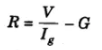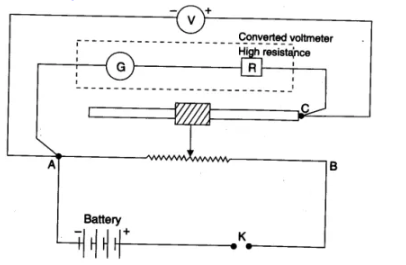# To Convert The Given Galvanometer (Of Known Resistance And Figure Of Merit) Into A Voltmeter Of Desired Range And To Verify The Same

## Aim:

To convert the given galvanometer (of known resistance and figure of merit) into a voltmeter of desired range and to verify the same.

## Apparatus Required:

A galvanometer, a voltmeter 0-3V, battery, 2 resistance box (10,000 ohms and 200 ohms), 2 one way keys, a rheostat, connecting wires and sandpaper.

## Theory:

A galvanometer is an ideal device that is capable of detecting even the weakest electric currents in an electric circuit. It features a coil suspended or pivoted between concave pole faces of a strong laminated horseshoe magnet. The galvanometer shows the deflection when an electric current is passed through the coil. The deflection is directly proportional to the current passed. A voltmeter is an instrument used for estimating the electrical potential difference between 2 points in an electric circuit.

### Series resistance required for conversionV-range of conversion

## Diagram## Procedure

1. Connect the resistance box in series combination with the galvanometer and take the plugs of resistance R.
2. A and B are the fixed terminals and C is the variable terminal of the rheostat.
3. Now the galvanometer functions as a voltmeter of range V Volts.
4. Take out the plugs of calculated resistance R from the resistance box.
5. By using a key, adjust the movable contact of the rheostat such that the deflection of the galvanometer becomes maximum.
6. Note both the readings of the galvanometer and voltmeter.
7. Convert the readings of the galvanometer into volts.
8. Find the difference in the reading. This difference between voltmeter reading and galvanometer reading gives the error.
9. By moving the variable contact of rheostat, take 5 readings covering the range of voltmeters from 0-3 Volts.

## Record your observation

The Least count of galvanometer converted into voltmeter = V/n =

 sl.no Reading of galvanometer into voltmeter Standard voltmeter reading V2 Standard voltmeter reading V2 – V1 deflection The potential difference in volts V1 1 2 3 4 5

## Calculation

The resistance of the given galvanometer G =

The figure of merit k =

Number of divisions in galvanometer scale n =

Current for full scale deflection = Ig = nk =

Range of conversion =

Resistance to be placed in series with galvanometer R=V/Ig-G =

## Precautions

• Calculate the resistance accurately
• Use the same range conversion voltmeter should be used for verification
• Use correct length shunt wire.

## Result

The value of the actual and measured value of the potential difference is very small and conversion is perfect.

## Viva Voce

1. On what principle does a galvanometer work?

Ans: Works on the principle of converting electrical energy into mechanical energy.

2. What is a voltmeter?

Ans: It is a device used to measure the potential difference between two points of the electrical circuit.

3. How is a galvanometer converted into a voltmeter?

Ans: A galvanometer is converted into a voltmeter by connecting it with high resistance.

4. Can a galvanometer be used to measure heavy currents?

Ans: No

5. What is the resistance of the ideal voltmeter?

Ans: infinite

## How can a galvanometer be converted into an ammeter?Test Your Knowledge On To Convert The Given Galvanometer Of Known Resistance

#### 1 Comment

1. Abhilash

It is the best learning center